X
Browse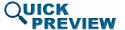• Abstract
• Authors
• Figures
• Multimedia
• References
• Cited By
• Keywords

# Spectrum Analysis Considerations for Radar Chirp Waveform Spectral Compliance Measurements

The measurement of a radar chirp waveform is critical to assessing its spectral compliance. The Fourier transform for a linear frequency-modulated chirp is a sequence of frequency-domain impulse functions. Because a spectrum analyzer measures the waveform with a finite-bandwidth intermediate-frequency (IF) filter, the bandwidth of this filter is critical to the power level and shape of the reported spectrum. Measurement results are presented that show the effects of resolution bandwidth and frequency sampling interval on the measured spectrum and its reported shape. The objective of the measurement is to align the shape of the measured spectrum with the true shape of the signal spectrum. This paper demonstrates an approach for choosing resolution bandwidth and frequency sampling interval settings using the example of a linear frequency-modulation (FM) chirp waveform.

SECTION I

## INTRODUCTION

The enforcement of spectral criteria in radar systems is significantly dependent upon the configuration used for spectrum measurement. Regulatory agencies must provide standards for the spectrum measurement. The international regulatory body for spectrum regulations is the International Telecommunication Union (ITU). Several ITU standards exist in describing allowable emissions and the measurement assessment of spectral compliance. Standard ITU-R SM.329 discusses allowable emissions in the spurious emission domain , and standard ITU-R SM.1541 describes allowable emissions in the out-of-band (OoB) domain, which is closer to the assigned bandwidth than the spurious domain . In light of these standards, an additional standard ITU-R M.1177-4 provides recommended settings for the measurement of emissions . The ITU Radio Regulations provide the spectral limitations of transmitted radio signals . In addition, individual nations have regulatory agencies that often expound upon the ITU standards for spectrum regulation. In U.S., the National Telecommunications and Information Administration (NTIA) sets guidelines for the spectrum properties in the radar spectrum emissions criteria (RSEC) . In addition to the RSEC, the NTIA has released a report by one of the authors detailing procedures for measurement assessment of RSEC compliance  and a report discussing receiver measurement bandwidth issues related to receivers . These criteria indicate measurement settings for evaluation of the spectrum and also bandwidth issues related to chirp waveforms for detrimental effects of interference. Among these criteria are maximum limits on the bandwidths that should be used to measure pulsed radar emission spectra. A topic not addressed in this existing literature, but which is addressed in this paper, is the justification for the maximum measurement bandwidth limits for linear frequency-modulation (LFM) waveforms, also known as “chirps.” While the ITU and NTIA standards provide recommendations for settings such as resolution bandwidth, our paper provides experimental data explaining the importance of these settings and connects the measurement science with the compliance-measurement recommendations. To our knowledge, this paper is the first to comprehensively address the issue of radar spectrum measurements for compliance from a measurement science perspective with experimental motivation and validation. We provide specific measurement examples of chirp waveforms and discuss, based on our experimental results, how changing the settings can adversely affect the measurement data, and possibly a compliance determination.

Linear chirps are useful radar waveforms because they provide improvement on range resolution for a given pulse length due to the ability to compress the pulse . In radar system operation, a burst of a sinusoid with increasing or decreasing frequency will happen during the radar's “transmit” time, and then the transmitter will hibernate during the “listen” time. The periodicity of the waveform produces a spectrum in which power is concentrated at a finite number of frequencies (a discrete spectrum).

The need for accurate and standardized spectrum measurement procedures is motivated by increasingly stringent spectral requirements on radar systems. In a struggling international economy, wireless broadband applications show promise in providing significant financial return. As such, new developments such as the U.S. President's Broadband Plan  are requiring that additional spectrum be allocated for wireless broadband use. Such developments are requiring radar transmitters to operate in narrower slices of spectrum. In the RSEC, the NTIA sets spectral masks in the U.S. within which radar signals are required to be confined , as shown in Fig. 1. Allocations are pushing the technical limits of operation for presently used legacy radar systems. The line of the mask shown in Fig. 1 allows a 40-dB bandwidth of 16 MHz; this means that at 8 MHz from the center frequency on each side, the signal must be reduced by 40 dB from its maximum in-band value. The mask then specifies that, outside of the 16 MHz bandwidth, the maximum signal level decreases at a rate of 20 dB per frequency decade away from the band.

Fig. 1. Spectral mask example, reprinted from . The mask requires the signal to be 40 dB below the maximum in-band level at 8 MHz from the center frequency, and the upper limit decreases at 20 dB/decade moving away from the band in both directions outside the 16 MHz bandwidth.

In addition to fixed measurement systems, standard methods of measurement will be essential in the real-time assessment and tuning of future adaptive radar systems –  . Our previous work has detailed a test platform  to perform such measurements and develop techniques with a goal of eventual integration into a real-time, adaptive radar system.

Some work exists in the open literature related to measurement evaluation of spectra for different applications. Engelson discusses the measurement of code-division multiple access (CDMA) signals in . Agilent Technologies  examines settings for measuring different types of signals and provides resolution and video bandwidth recommendations for different scenarios. Bertocco discusses the measurement of power using a spectrum analyzer from the “channel-power” and “zero-span” approaches, noting that resolution bandwidth in a channel-power approach should be narrow enough to resolve individual spectral components if a measurement of peak power is desired . He also notes that if a zero-span measurement of power is desired, the resolution bandwidth should be at least as large as the signal bandwidth to avoid underestimation of the signal power. While providing useful information about spectrum analyzer settings for different desired evaluations, Bertocco's treatment is focused on power measurements, rather than measurements to determine spectral compliance. In , Bertocco gives some theoretical considerations and discussion related to practical spectrum analyzer measurements, stating that several spectral components will fall into the filter passband simultaneously for the case of a line spectrum if the resolution filter bandwidth is wider than the spectral line separation, also discussed by . Bertocco et al.  also discusses the use of peak detectors to perform sampling. Multiple studies have also been performed on spectrum occupancy of different regional areas, with a view to the assessment of cognitive radio and dynamic spectrum access feasibility – . The challenge of setting a measured power threshold to label a region of spectrum as “occupied” is dealt with by Islam, who used a level of 6 dB over the minimum received power . Wellens states that a decision threshold of 3 dB higher than the measured noise floor was expected to result, in his study, in a false-alarm probability of about 1% . Sanders, in evaluating spectrum occupancy, suggests the use of resolution bandwidth equal to the span, allowing each spectral content to be represented exactly once in the measurement . This consideration of resolution bandwidth is one that we readdress in this paper, but with a view toward determining spectral compliance, a distinct application from other relevant papers.

The ITU standards also provide information related to measurements for spectral compliance. Standard ITU-R SM.329 describes reference bandwidth as the bandwidth in which the acceptable power is specified, and resolution bandwidth as the bandwidth used by the spectrum analyzer for measurement. This standard states that “narrower resolution bandwidth is sometimes necessary for emissions close to the center frequency.” ITU-R SM.1541  describes the assessment of emissions in the OoB domain, usually the region adjacent to the main channel that is affected by nonlinearity-induced intermodulation distortion. It defines the idea of adjacent-band power ratio as a useful measurement for assessing OoB power . Finally, ITU-R M.1177-4 recommends techniques for the measurement of the emitted spectrum based on  and . It also gives specific information on performing measurements close to the band of operation .

This paper presents the theory and measurement verification for the regulatory spectrum analysis of radar signals. It examines spectrum analysis considerations specifically for spectral compliance measurements. The work presented in this paper is significant because it provides a distinct look at the impact of spectrum analysis measurement considerations on spectral compliance evaluations. While the ITU standards provide some level of guidance on performing the measurements, the purpose of this paper is to provide understanding and experimental data to the measurement assessment of spectral compliance. This paper uniquely describes not only the “what” and “how” of spectrum measurements, but also addresses the question of “why” certain settings must be used to ensure accurate compliance determinations.

SECTION II

## CHIRP WAVEFORM ANALYSIS

During the “on” time of the transmitter pulse, a linear FM chirp has a linear time-frequency description. Fig. 2 depicts the frequency-versus-time characteristic. The frequency sweep begins at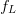$f_{L}$ and ends at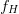$f_{H}$. As such the spectrum of the chirp will span the frequency range from$f_{L}$ to$f_{H}$. Because this pulsed chirp waveform is periodic, its spectrum will be discrete, consisting of impulse functions at integer multiples of the fundamental frequency. If the period of an entire on-off cycle of the chirp is given as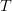$T$, then the fundamental frequency is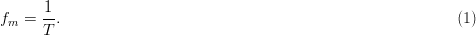TeX Source

Fig. 2. Frequency-versus-time characteristic of a linear-frequency modulated chirp. The period of the chirp is$T$ seconds, and μ/π is the slope of the chirp in Hertz per second.

As a result, the spacing between tones in the chirp spectrum is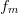$f_{m}$ .

Understanding that the spectrum of periodically repeated chirps is actually discrete is critical to the interpretation of measurement data in which the spectrum appears to be continous. This appearance does not represent the actual shape of the spectrum, but is an artifact of the spectrum analyzer measurement.

To illustrate an effective general measurement methodology, a simple case study is performed on measurements for a linear FM (LFM) chirp with 16 MHz swept bandwidth. We examine the same chirp for different measurement settings and assess potential issues in assessing the measurements, as well as using the chirp settings to plot a course for making desired measurements.

The complex exponential version of the LFM chirp waveform during the “on” time of the duty cycle is given as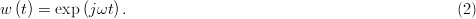TeX Source

The instantaneous frequency is a linear function of time and is expressed as follows, where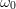$\omega _0$ is the radian frequency at$t$ = 0 and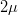$2\mu$ is the slope of the radian frequency-versus-time characteristic (this means that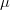${\mu}$/${\pi}$ is the Hertz frequency-versus-time slope)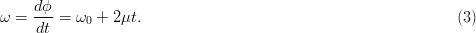TeX Source

The linear frequency-versus-time characteristic has a low-frequency limit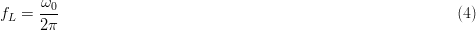TeX Source and high-frequency limit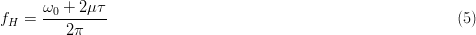TeX Source where${\tau}$ is the “on-time” pulse width of the chirp burst.

Table I provides information about the chirp settings for the running example used in this paper: 16 MHz chirp range, 3.3 GHz center frequency, and 30 kHz waveform repetition rate. For the purpose of the measurements presented, the chirp burst time τ was made to be equal to the chirp repetition time$T$; that is, a duty cycle of 100 percent was used for the measured waveform. The slope of the frequency-versus-time characteristic is then 2${\mu}$, where${\mu}$ is found by solving (5) for${\mu}$ using these settings, giving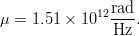TeX Source The linear chirp rate in Hertz per second is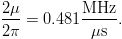TeX Source

Table I Chirp Parameters Used in this Experiment
SECTION III

## SPECTRUM ANALYZER MEASUREMENTS

A simplified block diagram model of a spectrum analyzer measurement is shown in Fig. 3 This diagram has been simplified to illustrate the functionality of systems important to these measurements; for a more detailed diagram, the reader is referred to . The signal to be measured is input to a mixer. A swept local oscillator is used to down-convert the measured RF signal to an intermediate frequency (IF). The IF resolution bandwidth filter, centered at the fixed IF, reports the power captured within its bandwidth and plots a representative point on the spectrum analyzer display. The swept oscillator is synchronized with the trace frequency on the spectrum analyzer display. The total power reported for a given frequency on the spectrum analyzer is the power that falls within the bandwidth of the IF filter.

Fig. 3. Spectrum analyzer simplified conceptual block diagram based on . The total power reported on the spectrum analyzer display is the total downconverted power that falls within the IF filter bandwidth.

The resolution bandwidth is of central importance in the measurement of a wideband signal, such as the LFM chirp. Because the signal is wideband, the power contained within the filter bandwidth increases as the bandwidth increases. This is underscored in the ITU standard ITU-R M.1177-4, which suggests for some simple modulations that the measurement bandwidth be less than the tone spacing, so that only one tone of the spectrum will be included in any measurement . The following describe different resolution bandwidth scenarios for measurement:

1. Narrow resolution bandwidth. If the bandwidth is sufficiently narrow, each of the discrete tones will be captured with a shape that is similar to the shape of the filter.
2. Wider resolution bandwidth. Wider resolution bandwidth may allow multiple tones to be captured inside the filter. If the filter bandwidth is wide enough to contain multiple tones in each measurement, then the total power will never reach zero.
3. Very wide resolution bandwidth. When the filter bandwidth is much larger than the tone spacing, the power measurement will produce a result that is nearly flat with frequency and has a power value consistent with the total power in the band at each measured frequency point.
SECTION IV

## MEASUREMENT RESULTS

This section evaluates the dependence of the measured spectrum upon resolution bandwidth and number of points used. It then outlines a recommended procedure for spectral compliance measurements.

### A. Effect of Resolution Bandwidth Setting

Measurements were performed with the spectrum analyzer to illustrate the dependence of the measurement data on the resolution bandwidth setting. The chirp waveform, with settings as shown in Table I, was generated in the laboratory using an Agilent N5182 MXG vector signal generator. To generate the waveform, the in-phase and quadrature component definitions of the waveform were created in MATLAB, and the waveform was created by the signal generator by playing the defined samples at a rate of 60 megasamples per second. The repetition rate of the entire chirp waveform is therefore dependent on the number of samples for one complete cycle of the chirp. For a chirp defined using 2000 samples and the instrument clock rate of 60 megasamples per second, the fundamental chirp repetition frequency is given by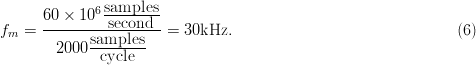TeX Source

Fig. 4(a) shows the resultant spectrum analyzer measurement with a narrow IF bandwidth. In this measurement, the individual tones are spaced by 30 kHz.

Fig. 4. Chirp measurement results for (a) 2000 samples at 60 megasamples per second, and (b) 500 samples at 60 megasamples per second.

A second measurement was taken with the same chirp rates, but with the waveform defined over only 500 samples. In this case, the fundamental chirp repetition frequency is given by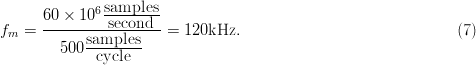TeX Source

Results for the measurement of this chirp are shown in Fig. 4(b). In this case, the tones are spaced by 120 kHz, as expected. The remainder of the experimental results shown in this paper is for 30 kHz tone spacing [as in Fig. 4(a)], given by Table I.

Fig. 5 shows measurement results for three resolution bandwidth values for spectral tone spacing (fundamental frequency) of 30 kHz.

1. Resolution Bandwidth Smaller Than the Tone Spacing (10 kHz) : Fig. 5(a) shows the results for 10- kHz resolution bandwidth. In this case, the resolution bandwidth is significantly smaller than the tone spacing, so no more than one tone will be present in the measurement IF bandwidth at any measurement instant.
2. Resolution Bandwidth Equal to the Tone Spacing (30 kHz): Fig. 5(b) gives measurement results for a resolution bandwidth of 30 kHz. In this case, the resolution bandwidth is equal to the separation of the tones to be measured, so as one tone leaves the measurement bandwidth, another enters. The nulls between the measured peaks for a resolution bandwidth of 30 kHz are not as deep as the nulls for a resolution bandwidth of 10 kHz.
3. Resolution Bandwidth Greater Than the Tone Spacing (100 kHz): Fig. 5(c) shows the measured spectrum power for 100 kHz resolution bandwidth. In this case, the measurement bandwidth is much wider than the tone separation. The IF filter contains at most four tones and at least three tones for each measured data point. The variation between the Watt power in a bandwidth should not be more than 25 percent of the maximum. This corresponds to an expected variation that is within approximately +/−1.25 dB. Fig. 5(c) shows a variation on this order. The slight variation is based on whether three or four tones are within the resolution bandwidth at each measured point.
Fig. 5. Chirp spectrum analyzer measurements for resolution bandwidth values of (a) 10 kHz, (b) 30 kHz, and (c) 100 kHz.

As the resolution bandwidth is increased, the variation between measured points will decrease even further. The ripple versus frequency as the filter moves to measure each point reduces as the number of tones in the bandwidth becomes larger. Fig. 6 shows the results for a broader frequency span, revealing the measurement of the entire chirp spectrum, using resolution bandwidths of 100, 5, and 1 kHz.

1. Large (100 kHz) Resolution Bandwidth: For 100 kHz resolution bandwidth [see Fig. 6(a)], the power value in the band is much larger, the in-band characteristic is very flat, the apparent bandwidth is much larger than the chirp bandwidth of 16 MHz, and the OoB degradation is gradual. The large resolution bandwidth for the 100 kHz setting allows multiple tones to appear within the bandwidth at each measured point, causing the measured power level to appear very high (−19.1 dBm) in the band. This also accounts for the gradual OoB degradation, as even with a center frequency out of the band of the chirp, multiple tones from inside the chirp bandwidth may still appear within the filter's resolution bandwidth.
2. Medium (5 kHz) Resolution Bandwidth: Fig. 6(b) shows the same 16 MHz chirp measured with a resolution bandwidth of 5 kHz, one-twentieth the size of the previous case. The in-band measurement shows small ripples, the apparent chirp bandwidth is narrower, the measured power in the band is significantly lower (−37.82 dBm), and the OoB rolloff is smoother and much quicker.
3. Small (1 kHz) Resolution Bandwidth: Fig. 6(c) shows the results for an even narrower resolution bandwidth (1 kHz), and it can be seen that the measured in-band power is approximately −40 dBm. A reduced noise floor is also evident. Because the measured noise power is equal to kTB, where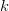$k$ is Boltzmann's constant,$T$ is the Kelvin temperature, and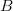$B$ is the Hz bandwidth, the noise floor is expected to decrease as the resolution bandwidth is decreased. This shows details in the actual spectral spreading that would be lost by a measurement with wider resolution bandwidth.
Fig. 6. Spectrum analyzer results for resolution bandwidths of (a) 100 kHz, (b) 5 kHz, and (c) 1 kHz.

ITU standard ITU-R M.1177-4 specifically refers to the situation shown in the following passage found in the standard :

“Measurements should generally be made using a bandwidth that is close to but less than the specified reference bandwidth. This approach will minimize the measurement time but it also causes some broadening of the measured spectrum. Thus in marginal situations, where measurement of the true close in spectrum shape may be important, it is recommended that the close-in region within the OoB domain should be measured using a maximum bandwidth of 0.2/T or 0.2/t as appropriate.”

The “broadening of the measured spectrum” is seen when a large measurement spectrum is used, such as in Fig. 6(a). In the case of this chirp, the tone spacing is 30 kHz (this is 1/$T$), so the recommended measurement bandwidth based on this ITU-R M.1177-4 passage is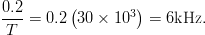TeX Source

This means that both Fig. 6(b) (resolution bandwidth = 5 kHz) and (c) (1 kHz) are acceptable in regard to limitation of spectral spreading for compliance purposes. However, the smaller resolution bandwidth in the measurement of Fig. 6(c) has a lower noise floor, which may be necessary if the spectral mask evaluation requires measurement of low power levels.

### B. Effect of Measured Points Number

Fig. 7 shows the same chirp as is displayed in Fig. 6, measured with a varying number of points. Fig. 7(a) shows the results for 401 points, Fig. 7(b) shows results for 1601 points, and Fig. 7(c) shows the measured spectrum for 6601 points. No in-band null points are seen in Fig. 7(a), but a significant number of nulls is visible in the measurement of Fig. 7(b), and Fig. 7(c) shows an even greater number of nulls.

Fig. 7. Spectrum analyzer measurements of chirp waveform for a resolution bandwidth of 1 kHz and (a) 401 points, (b) 1601 points, and (c) 6601 points.

An understanding of the video stage of the spectrum-analyzer front-end, as described in a very useful Agilent application note , assists in interpreting these results. The local oscillator is tuned by the voltage output of a sweep generator. As a result, spectral content over the range of RF frequencies to be measured is downconverted and swept through the resolution-bandwidth filter at the IF.

The signal is then passed through an envelope detector, which provides a measurement of the power inside the filter. The video bandwidth filter follows, followed by a peak-sample-hold circuit. When the spectrum analyzer is in peak measurement mode (as for the results shown in this paper), this circuit reports the peak value of the sweep surrounding each measured point on the spectrum analyzer screen.

### 1. Small Number of Measured Points (401)

For all three measurements shown in Fig. 7, the span can be seen to be 100 MHz (3.25−3.35 GHz). For the 401-point measurement of Fig. 7(a), a total of 401 points was used. This means that the frequency spacing between measured data points, and equivalently, the frequency interval over which a peak value is sampled, here called Δ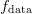$f_{\rm data}$, is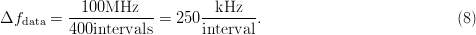TeX Source

Thus, for each measured data point, an interval of 250 kHz is swept with a resolution bandwidth of 1 kHz and the peak value reported through the “sample-and-hold” approach. The results are connected on the spectrum analyzer output. Because the tones for this measurement are spaced by$f_{m}$ = 30 kHz, at least eight tones appear in each sampled range. The maximum value measured for these tones will be recorded. This is why no nulls appear in the 401-points result of Fig. 7(a).

### 2. Moderate Number of Measured Points (1601)

For the measurement of Fig. 7(b), a total of 1601 points was used. This means that the value for Δ$f_{\rm data}$ is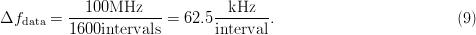TeX Source

Because the tone spacing is 30 kHz, it is expected that most intervals will contain at least two tones. Because the resolution bandwidth is narrow, however, the power levels reported at the points are expected to be the similar to the 401-point measurement of Fig. 7(a). The plot of Fig. 7(b) contains more noise, as the maximum in each region is taken over only two measured tones, rather than 8. This increases the variation of the maximum-value measurements from point to point.

### 3. Large Number of Measured Points (6601)

Fig. 7(c) contains the largest number of null points. In this case, the value of Δ$f_{\rm data}$ is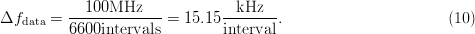TeX Source

For a tone spacing of 15.15 kHz, the probability that a tone will be detected in a given sweep range is approximately only 50%, and so the number of zero measurements, or “nulls,” is expected to be significant. Commensurate with this expectation, Fig. 7(c) shows a measurement containing many zeros.

While it is interesting from a spectrum analyzer to see the locations of the tones and nulls, the identification of individual tones and their locations is not critical in the measurement of chirps for spectral compliance assessment. The outline of the spectral shape is more clearly seen in the graph of Fig. 6(a) than in Fig. 6(b) and (c). The shape is most easily and accurately seen when the number of points is small enough that at least one tone is contained in each measurement window. Optimally, the resolution bandwidth will be small enough that the IF filter passband never contains more than one tone at any point during the measurement. Measurements such as those shown in Fig. 6(c) and 7(a) are best for assessing compliance with RSEC or other spectrum evaluation criteria.

SECTION V

## GUIDELINES FOR SPECTRAL COMPLIANCE MEASUREMENTS

As shown in the measurement results, the resolution bandwidth and number of points have a significant impact on the measured data. How the data are measured can have an impact on whether the signal appears to meet the spectral mask requirements or to fail compliance. Based on the experiments shown, some general guidelines for spectral compliance measurements are given in this section. For all measurements, the span should be set large enough to view the desired channel and adjacent channels under consideration.

### A. Resolution Bandwidth

If possible, the resolution bandwidth should be set to a small enough value that only one tone is present in the resolution bandwidth for each measurement. In most cases, this is several orders of magnitude smaller than the assigned channel bandwidth. The measurement of power at each prespecified frequency is the total power in the resolution bandwidth centered around this frequency. As such, a small bandwidth should be used surrounding each measurement point.

The danger of setting the resolution bandwidth too large is that power will be reported at a given frequency that is actually from spectral content a significant distance from that frequency. This is referred to by ITU-R M.1177-4 as “broadening of the measurement spectrum,”  and could result in an improper determination of spectral compliance. Fig. 6(a) is an example of a measurement where this undesirable effect occurs. A resolution bandwidth of 100 kHz was used for this measurement, meaning that spectral content is included at each measured point for 50 kHz on both sides of the reported frequency. Because the repetition frequency of the chirp in these measurements is 30 kHz, this means that either 3 or 4 tones will be present in the resolution bandwidth for each measurement. This causes apparent “spreading” of the signal, and could cause a radar system to be declared as noncompliant, when, in fact, its power actually resides within the spectral mask. An erroneous declaration of noncompliance could result in the prevention of a compliant system from being put into operation at great cost to both the manufacturer and the end user. For ideal spectral compliance measurements, the resolution bandwidth should be less than the tone spacing (in the case of the experiments shown, less than 30 kHz). ITU-R M.1177-4 recommends that an even smaller value of 0.2/$T$ should be used, where$T$ is the period of the waveform . This corresponds to 6 kHz for Fig. 6 example.

A second artifact of using a smaller resolution bandwidth is that it causes the noise floor to be lower. ITU-R M.1177-4 states “To obtain a complete picture of the spectrum especially in the spurious emission domain, it is recommended to be able to measure levels of emissions 10 dB below the levels given in RR (ITU Radio Regulation) Appendix 3” . The spurious emission domain contains frequencies some distance away from the main channel, and these are usually required to have much lower power values than the in-band signal. For example, if the signal is required to be 50 dB down from the maximum in-band power at the alternate channel, but the spectrum analyzer measurement shows a noise floor that is only 40 dB down from this maximum in-band power, mask compliance cannot be assessed. Based on ITU-R M.1177-4, the noise floor must be reduced by 20 dB to give 10 dB between the mask value and the noise floor. From noise measurement theory, the noise floor changes based on the resolution bandwidth as follows :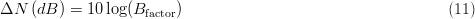TeX Source where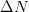$\Delta N$ is the change in dB of the noise floor, and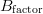$B_{{\rm factor}}$ is the factor by which the resolution bandwidth is multiplied. Solving for$B_{{\rm factor}}$ for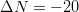$\Delta N = - 20$ dB gives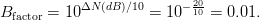TeX Source

Thus, reducing the resolution bandwidth by a factor of 100 will provide a noise floor reduction of 20 dB.

If the resolution bandwidth is very small, the sweep time will be very large, and taking the data necessary for the measurements will become an inefficient process. Thus, the resolution bandwidth should simply be set low enough so that (1) only one tone exists in the resolution bandwidth filter for each measurement and (2) the measurement noise floor is low enough to assess the compliance for the OoB frequencies of interest. However, requiring the resolution bandwidth to be lower than this will unnecessarily slow the measurement.

### B. Number of Measured Points

The tones in a spectrum are spaced by the repetition rate of the radar chirp. If peak measurement mode is used, then as long as the measured points are spaced apart by a larger frequency than the waveform's repetition rate, a tone will be seen in each frequency range corresponding to a measured point, and a continuous trace will be viewed on the screen, rather than a line spectrum. This improves the ease of viewing the mask compliance, but offers little risk of error in either improperly declaring a radar in compliance or out of compliance. It also speeds the measurement, as a larger number of measured points requires more time.

SECTION VI

## CONCLUSION

A spectrum analyzer, with a heterodyne receiver in its front end, measures spectrum analysis data by relocating different frequency ranges to pass through the resolution bandwidth filter at the IF. Small resolution bandwidth is best for accurate assessments of spectral compliance. Large resolution bandwidth causes the bandwidth of the chirp to appear too large. Closely spaced tones can be discerned only by performing a low-resolution-bandwidth measurement with enough measured frequency points that the frequency separation between measured points is smaller than the Fourier-series tone spacing. In the case where this separation is large, zero measurements, i.e., nulls, can be identified between tone locations. For spectral compliance assessment, identification of these nulls is not important; the envelope of the spectral peaks is much more useful in assessing spectral compliance. Based on these considerations, the number of points used should be small enough that at least one tone is measured in each data range, but large enough to show the shape of the spectrum correctly. The ITU standards suggest a resolution bandwidth smaller than the tone spacing for chirp signals, and the suggestions of these standards have been demonstrated to be useful through the experimental data we have presented.

The results of this study provide an understanding of measurement considerations related to spectral compliance assessment for radar transmitters and other similar broadcasters of wideband signals.

### Acknowledgement

The authors would like to thank Dr. E. Mokole of the Naval Research Laboratory for his helpful guidance of this work, as well as Baylor research assistants M. Fellows, D. Moon, and O. Akinbule for their help. Special thanks also goes to Dr. L. Dunleavy and Dr. T. Weller of the University of South Florida for the conceptual block diagram concept of Fig. 3. We wish to thank the reviewers of this paper for their suggestions; their expertise, and input has resulted in a much more useful and improved contribution to the EMC field.

## Footnotes

This work was supported by a grant from the U.S. Naval Research Laboratory. C. Baylis, J. Martin, M. Moldovan, and R. J. Marks, II, with the Electrical and Computer Engineering, Baylor University, TX 76706 USA (e-mail: Charles_Baylis@baylor.edu; Josh_Martin@baylor.edu; Matt_Moldovan@baylor.edu; Robert_Marks@baylor.edu).

None

## Corrections

None

This paper appears in:
Electromagnetic Compatibility, IEEE Transactions on
Issue Date:
June 2014
On page(s):
520 - 529
ISSN:
0018-9375
INSPEC Accession Number:
None
Digital Object Identifier:
10.1109/TEMC.2013.2291540
Date of Current Version:
2014-05-21
Date of Original Publication:
2013-12-03

## Need Help?

• US & Canada: +1 800 678 4333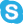+1 800 678 4333
• Worldwide: +1 732 981 0060+1 732 981 0060 FREE
• Contact & SupportAbout IEEE Xplore | Contact | Help | Terms of Use | Nondiscrimination Policy | Site Map | Privacy & Opting Out of Cookies

A not-for-profit organization, IEEE is the world's largest professional association for the advancement of technology.